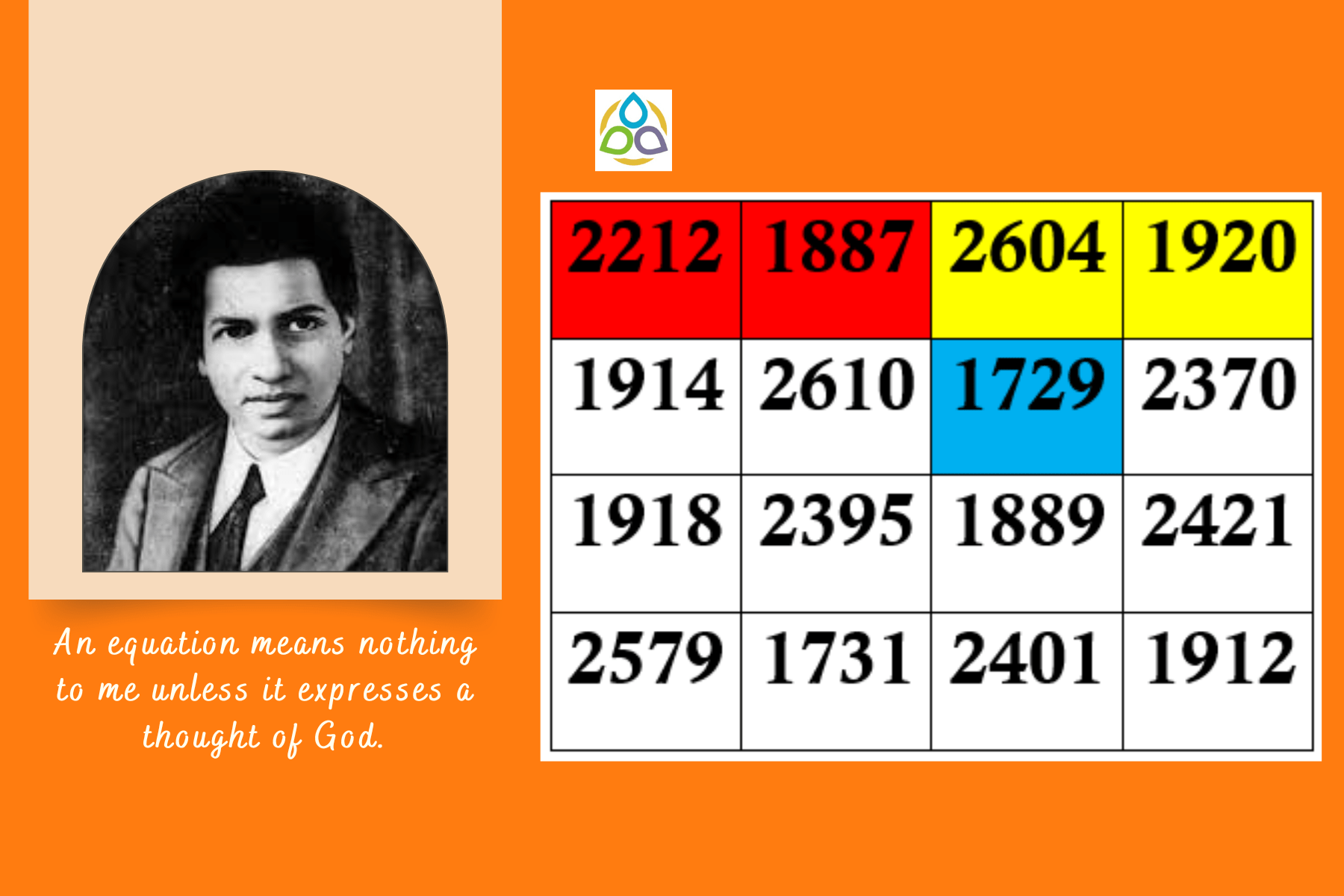## Remembering Ramanujan: A Magic Square Tribute on His Death Anniversary

While asleep, I had an unusual experience. There was a red screen formed by flowing blood, as it were. I was observing it. Suddenly a hand began to write on the screen. I became all attention. That hand wrote a number of elliptic integrals. They stuck to my mind. As soon as I woke up, I committed them to writing.

Srinivasa Ramanujan

Srinivasa Ramanujan was a renowned Indian mathematician who made significant contributions in several fields of mathematics. Since childhood, Ramanujan had a fascination with numbers and magic squares. In honor of his birthday, there is a magic square that bears his name. He is most famously associated with the number 1729, which is now known as the Hardy-Ramanujan number. Ramanujan passed away on April 26, 1920, at the young age of 32.

In this blog post, we will consider a magic square of order four that commemorates his death anniversary as well. The top row of the square is filled with numbers derived from Ramanujan’s birthdate (December 22, 1887) and his death date (April 26, 1920). One cell in the second row also uses the number 1729 in honor of Ramanujan. Additionally, the magic sum of the square is 8623, which is a prime number.

## Few Notable Properties

(a) The sum of the row elements in each row is equal to 8623. For example,

2212 + 1887 + 2604 + 1920 = 8623

1914 + 2610 + 1729 + 2370 = 8623

1918 + 2395 + 1889 + 2421 = 8623

2579 + 1731 + 2401 + 1912 = 8623

(b) The sum of the column elements in each column is equal to 8623. For example,

2212 + 1887 + 2604 + 1920 = 8623

1914 + 2610 + 1729 + 2370 = 8623

1918 + 2395 + 1889 + 2421 = 8623

2579 + 1731 + 2401 + 1912 = 8623

(c) The sum of the diagonal elements is also equal to 8623. For example,

2212 + 2610 + 1889 + 1912 = 8623 (green)

1920 + 1729 + 2395 + 2579 = 8623 (light blue)

(d) The sum of the corner elements is also equal to 8623. For example,

2212 + 1920 + 1912 + 2579 = 8623

(e) The sums of the numbers in the two sets of like coloured cells are again the same number 8623. For example,

1887 + 2604 + 2401 + 1731 = 8623 (yellow)

1914 + 1918 + 2421 + 2370 = 8623 (purple)

(f) The sums of the numbers in the two sets of like coloured cells are again the same number 8623. For example,

1887 + 1914 + 2401 + 2421 = 8623 (yellow)

2604 + 2370 + 1731 + 1918 = 8623 (blue)

(g) The sum of the numbers in the four central cells is again 8623. For example,

2610 + 1729 + 1889 + 2395 = 8623

(h) The sums of the numbers in the like-coloured 2 × 2 blocks are all 8623. For example,

2212 + 1887 + 2610 + 1914 = 8623 (light blue)

2604 + 1920 + 2370 + 1729 = 8623 (orange)

1889 + 2421+ 1912 + 2401 = 8623 (olive green)

1918 + 2395 + 1731 + 2579 = 8623 (green)

Your suggestions are eagerly and respectfully welcome! See you soon with a new mathematics blog that you and I call Math1089 – Mathematics for All!“.